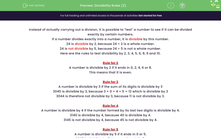# Know The Rules for Divisibility

In this worksheet, students will use their knowledge of all the divisibility rules to state by which numbers the given numbers are divisible.Key stage:  KS 3

Curriculum topic:   Number

Curriculum subtopic:   Use Concepts and Vocabulary for All Numbers

Popular topics:   Ratio worksheets

Difficulty level:#### Worksheet Overview

Instead of actually carrying out a division, it is possible to test a number to see if it can be divided exactly by certain numbers.

If a number divides exactly into a number, it is divisible by this number.

24 is divisible by 2, because 24 ÷ 2 is a whole number.

24 is not divisible by 5, because 24 ÷ 5 is not a whole number.

Here are the rules to test divisibility by 2, 3, 4, 5, 6, 8, 9 and 10.

Rule for 2

A number is divisible by 2 if it ends in 0, 2, 4, 6 or 8.

This means that it is even.

Rule for 3

A number is divisible by 3 if the sum of its digits is divisible by 3

3,045 is divisible by 3 because 3 + 0 + 4 + 5 = 12 which is divisible by 3

3,044 is therefore not divisible by 3 because 11 is not divisible by 3.

Rule for 4

A number is divisible by 4 if the number formed by its last two digits is divisible by 4.

3,140 is divisible by 4 because 40 is divisible by 4.

3,145 is not divisible by 4 because 45 is not divisible by 4.

Rule for 5

A number is divisible by 5 if it ends in 0 or 5.

3,045 is divisible by 5 because it ends in 5.

3,030 is divisible by 5 because it ends in 0.

5,551 is not divisible by 5.

Rule for 6

Both the rules for 2 and 3 must work.

Rule for 8

A number is divisible by 8 if the number formed by its last three digits is divisible by 8.

3,240 is divisible by 8 because 240 is divisible by 8.

3,145 is not divisible by 8 because 145 is not divisible by 8.

Rule for 9

This is similar to the rule for 3.

A number is divisible by 9 if the sum of its digits is divisible by 9

7,065 is divisible by 9 because 7 + 0 + 6 + 5 = 18 which is divisible by 9

3,045 is therefore not divisible by 9 because 12 is not divisible by 9.

Rule for 10

A number is divisible by 10 if it ends in 0.

3,140 is divisible by 10 because it ends in 0.

3,145 is not divisible by 10.

Rule for 11

A number is divisible by 11 if alternate digit sums differ by 0 or a multiple of 11

Example

Is 3,813 divisible by 6?

No, because 3 + 8 + 1 + 3 = 15, which is divisible by 3, but it is not divisible by 2 as it ends in a 3 which is odd.

Example

Is 2,153,864 divisible by 4?

Yes because 64 is divisible by 4.

Example

Is 17226 divisible by 11?

Underline alternate numbers to get 17226

Add up the underlined digits to get the sum of 1 + 2 + 6 = 9

Add up the non-underlined digits to get the sum of 7 + 2 = 9

These two digit sums are equal or differ by a multiple of 11, so 17,226 is divisible by 11.

There's lots to remember here, so look back at the introduction at any point to check out the rules. You can do this by clicking on the red button at the side of the screen.

### What is EdPlace?

We're your National Curriculum aligned online education content provider helping each child succeed in English, maths and science from year 1 to GCSE. With an EdPlace account you’ll be able to track and measure progress, helping each child achieve their best. We build confidence and attainment by personalising each child’s learning at a level that suits them.

Get started